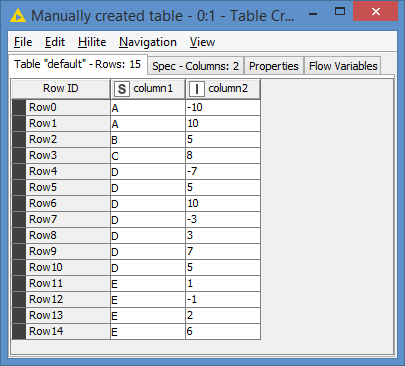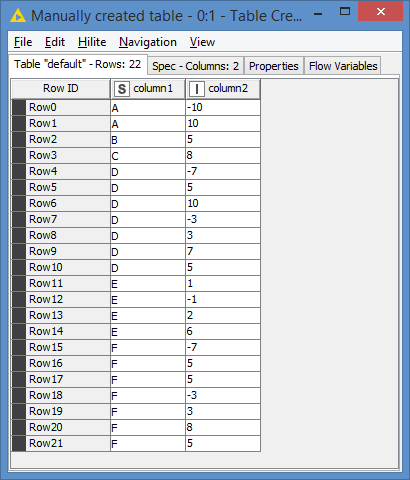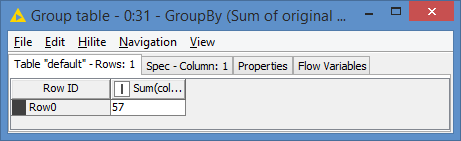# Eliminate rows that values in a column that sums up to zero

hello everyone im new user in knime, in one column i have values negative and positive, the achivement is clear that values that sums up to zero, an example like this :
-10
10
5
8
-7
-3
10
i have found the way to solve it only with the values that are exactly the same like in the two first columns, but if you realized the three last rows sums it will be zero, could you help me.

Hello @Angel_Villa,

and welcome to KNIME Community!

Not sure I understand. What is the desired output from above input?

Br,
Ivan

2 Likes

I have an input like this:
A -10
A 10
B 5
C 8
D -7
D -3
D 10
D 5
D 3
E 1
E -1
E 2
E 6
I want the output like this:

B 5
C 8
D 5
D 3
E 2
E 6

In the groups are some numbers than can be sum and automaticalli are eliminate, but i want to mantain the others, in the example that occurs with the group “D and E”.

1 Like

Would the numbers that per group (A, B,C,D) would add up to 0 always occur in a row or can they just be mixed up and the system would have to sort the ones out that can sum up to 0.

In fact they just be mixed up and the system have to sort the ones out that can sum up to 0.

Hi @Angel_Villa and welcome to the Knime Community.

The sample data you provided is a bit too simple and does not cover different scenarios, so it’s hard to know what to implement.

For example, see the following data:If you look at the values for D:
We have the same values as per your original data, I just switched some value around, but I also introduced 2 additional rows for D, with values 5 and 7.

So from your original data, your result was that -7 -3 +10 = 0.

From my data, you now have 3 possibilities to “counter” the -7-3:
-7-3+10
-7-3+3+7
-7-3+5+5

All 3 combinations give 0. How do we choose which combination should be used? Or does it matter which one to choose?

Also in your example, the “counter” value was right before or after the negative value(s), that is why I shuffled around my values. Are the counter values always supposed to be around the negative values (and hence that’s how we would choose which combination to use), or they could be all over the place like in my sample?

And lastly, do you always have the counter values? For example, can you end up with this data?
D -7
D -3
D 5
D 3

In this case, there aren’t the proper counter values, you will end up with:
D -7
D 5

Is that possible?

It does not matter the combination, but always it belong to the same group (example D,E) and exists into the group only one exact combination to sum up zero, and yes always has the “counter” values, the target is to eliminate the negatives.

Here is the example, all the negatives is equal than one positive.

Hello @Angel_Villa,

under assumption that in one group all negative numbers must sum up to one value this simple workflow should do the trick:Here is workflow.
2021_10_21_Summing_Up_Zero.knwf (24.1 KB)

If above assumption is wrong then attached workflow is no good. In that case can you modify input data in it so we can see other possible cases?

Br,
Ivan

2 Likes

Hi @ipazin , I think your solution is incomplete… The matching of the sum of the negatives do not necessarily match to 1 positive only. In some cases, it could need combinations of positives, which is the tricky part. For example, -7-3 could need to match with 5+5. So that means you can’t join directly to the results of the positives, but rather to a combination of them.

EDIT: At least that is what I understood when I asked about it. If @Angel_Villa can confirm that the sums of the negatives would match to only 1 positive, that would be a much easier solution - it would basically be what you provided.

Hello @bruno29a,

just wanted to cover basic case which satisfies given examples (no case where “-7-3 could need to match with 5+5” in them) cause anything else seems complicated at first and perhaps not necessary. Also if not mistaken this is financial data where e.g. positive numbers are budgets while negative numbers are actuals and if what you say is possible then there is no way to resolve 5 5 5 -5 -5. Or at least no way without additional information. What I’m saying there must be a logic behind these numbers and not all combinations are possible. Are you maybe a mathematician?Br,
Ivan

1 Like

Hi @ipazin , I actually do have a degree in MathematicsBut you don’t need to be a mathematician for what we are dealing with here. You learn this when you’re like 8-10 years oldA 10
A -7
A -2
A -1
A 12
A -8
A -4

While -7, -2 and -1 are countered by only 1 positive, which is 10, and similarly -8 and -4 are countered by only 1 positive, which is 12, this type of situation does not fall into the “basic” case and would not work, since in reality, we would have a total of -20, and the basic case would try to look for a positive 20.

Hopefully that is also clear for everyone, especially @Angel_Villa

1 Like

Hey @bruno29a,

asked regarding Mathematics cause the way you think and not so related to this topic. Actually also have a degree in MathematicsBut again this case is not in examples @Angel_Villa has given and my assumption (stated above) excludes this caseBr,
Ivan

3 Likes

Hi all,

I guess what @ipazin was wondering here is whether you were a mathematician rather than an accountant, since this sounds an accountant problem. He was not questioning your mathematics lolMy two cents on mathematics : we are here again face to a non-well-posed problem, as in other occasions. In the case here, the solution exists but as @ipazin mentioned, it may not be unique. Thus, he has just suggested a possible “regularized solution” which adds a condition and constrains the solution to be unique.

As you said @bruno29a,

… but he needs to clarify it.

Best

Ael

PS: Btw this is definitely and inverse problem2 Likes

Hi @ipazin @aworker , while we’re waiting for confirmation from @Angel_Villa , I thought that finding combinations for the positives was quite an interesting challenge.

So, I put something together for this - note, it will also work if no combination is needed, which basically is a combination of 1 item, so it will still work.

I made use of Python, since the Python script since I it has a library to generate all combinations of a given list.

The workflow looks like this:

This is actually a v1.1 of my workflow - I’ll explain what’s the difference between 1.0 and 1.1.

Before going into details, here’s the results.
First, the input data:Results:The difference between v1.0 and v1.1 relates to the case of F. When I built v1.0, the approach was that the Python script would retrieve the combinations for me, and then in Knime, I would exclude the rows whose values are in the combination. This works, however, the issue is that when you have the value more than once in the column, then you are in trouble.

For example, say you have something like this:
Z -10
Z 7
Z 3
Z 3

The combination correctly returns [7,3]. However, when trying to exclude 7 and 3, it would actually exclude all rows with 7 and all rows with 3. I could not find a way in Knime to do a row filter but only on 1 occurrence.

But it’s also a bit more complicated than that. For example, let’s say I have this case (and that’s exactly what F is):
F -10
F 5
F 5
F 5

The combination correctly returns [5,5]. So not only I want to find only 1 occurrence, but it has to be 1 occurrence per element of the combination. So I need 1 occurrence of 5 (the first 5), and 1 occurrence of 5 (the second 5).

As a result, I actually implemented the removal of the elements directly in Python, because the method list.remove() allows you to remove only 1 occurrence of it.

Of course, the best way to check that the workflow works is to check the sum of the original data and sum of the processed data (they should both be the same):

Original sum:Sum after process:Here’s my workflow: Eliminate rows that sums up to zero.knwf (47.6 KB)

4 Likes

Thanks , your solution was so creative, it works well.

2 Likes

Using Python is like buying dough when doing pizzaIvan

1 Like

lol @ipazin , I’m not a Python dev and indeed it’s a good analogyBut I can’t see any other way apart from using a script to accomplish the removal of only 1 instance of an element.

2 Likes

Well @bruno29a I saw you like analogies so I tried one myselfCan Duplicate Row Filter maybe help?
Ivan

It was a good one @ipazinDuplicate Row Filter would not work here, as there are exceptions that would not work.

1 Like